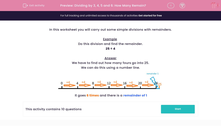# Divide by 3, 4, 5 and 6 with Remainders

In this worksheet, students will carry out simple divisions of two-digit numbers and note the remainders.This content is premium and exclusive to EdPlace subscribers.Key stage:  KS 2

Curriculum topic:   Maths and Numerical Reasoning

Curriculum subtopic:   Mixed Problems

Difficulty level:#### Worksheet Overview

In this activity, we will carry out some simple divisions with remainders.

e.g. Calculate this division and find the remainder:

25 ÷ 4

We have to find out how many fours go into 25.

We can do this using a number line.It goes 6 times and there is a remainder of 1.

Let's try some questions now.

### What is EdPlace?

We're your National Curriculum aligned online education content provider helping each child succeed in English, maths and science from year 1 to GCSE. With an EdPlace account you’ll be able to track and measure progress, helping each child achieve their best. We build confidence and attainment by personalising each child’s learning at a level that suits them.

Get started